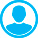APPROXIMATION APPROACH TO THE SOLUTION OF GRAVITY AND MAGNETIC PROBLEMS. 2. NEW METHODS OF GETTING STABLE APPROXIMATE SOLUTIONS OF LINEAR ALGEBRAIC EQUATIONS WITH THEIR APPROXIMATED RIGHT SIDESAbstract (English):
The abstract of this paper was not submitted. It is the continuation of paper "Approximation approach to the solution of gravity and magnetic problems. 1.The regularization of the systems of linear algebraic equations as the main computation problem" by V. N. Strakhov and A. V. Strakhov

Keywords:
gravity and magnetic problems, approximation approach, regularization of algebraic equations.